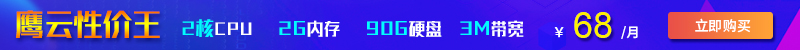Python中怎样利用while求1到100的和？这篇文章给大家分享几个Python中利用while循环实现求和的示例，下面介绍了用while求1到100的和、1到100内的偶数和、1到100内的奇数和文中示例代码介绍的非常详细，具有一定的借鉴价值，感兴趣的朋友可以参考一下，下面我们一起来学习一下吧。

## 1、1到100的和

定义2个变量i和`sum`，初值都为1，i的值每次增加1,取到100后结束程序，`sum`的值等于自身再加上i的值。这样i从2取到100，并且每次都与sum相加。

```#!/usr/bin/env python
#-*- coding:utf-8 -*-
i=1
sum=1
while True:
i+=1
sum=sum+i
if i==100:
break
print(sum)

```

## 2、1到100内的偶数和

```#!/usr/bin/env python
#-*- coding:utf-8 -*-
i=0
sum=0
while True:
i+=2
sum=sum+i
if i==100:
break
print(sum)

```

```#!/usr/bin/env python
# -*- coding:utf-8 -*-
# Python学习交流群：778463939
num=0
sum=0
while True:
num+=1
if num%2==0:
sum=sum+num
if num==100:
break
print("Task finished!The sum of even numbers from 1 to 100 is:  "+str(sum))

```

## 3、1到100内的奇数和

```#!/usr/bin/env python
#-*- coding:utf-8 -*-
i=int(1)
sum=int(1)
while True:
i+=2
sum=sum+i
if i==99:
break
print(sum)

```

```#!/usr/bin/env python
# -*- coding:utf-8 -*-
num=0
sum=0
while True:
num+=1
if num%2==1:
sum=sum+num
if num==100:
break
print("Task finished!The sum of odd numbers from 1 to 100 is:  "+str(sum))
```

以上就是Python中利用while求1到100的和的代码，需要的朋友可以参考，本文对大家学习while循环的使用有一定的帮助，希望大家阅读完这篇文章能有所收获。想要了解更多while循环的使用，大家可以关注其它的相关文章。

2022-12-14 18:25:53

2022-11-02 18:18:37

2022-07-09 17:35:45• 联系我们
• 24小时售后：4006784567
• 24小时TEL ：0668-2555666
• 售前咨询TEL：400-678-4567
•官方微信官方微信
Copyright  ©  QY  Network  Company  Ltd. All  Rights  Reserved. 2003-2019  群英网络  版权所有   茂名市群英网络有限公司